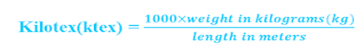top of page
Search

# Yarn count

Updated: Oct 24, 2021

Count of yarn:

A count is a number that represents the linear density i.e., length/weight or weight/length of the yarn and it also represents the fineness, coarseness, or size of the yarn.

Types of yarn count:

The count can be classified into two types of count systems based on natural and manmade fiber.

They are

• Direct count system(W/L)

• Indirect count system(L/W)

Direct count system(W/L):

A direct count system is used to measure the count for artificial fiber. In this system length is constant and for higher count numbers coarser will be the yarn and smaller count numbers yarn will be finer.

There are different systems that can be used in a direct count system.

They are

• Tex

• Decitex

• Milli tex

• Kilo tex

• Deniar

• Spyndle count

• Grex countTex:

It represents the weight in grams of 1000 meters of yarn.

For example, 1 Tex indicates that there is 1 gram of yarn per 1000 meters of that yarn.

1 Tex: 1000 meters of length and 1 gram of weight

10 Tex: 1000 meters of length and 10 grams of weight

20 Tex: 1000 meters of length and 20 grams of weight.Decitex(d.tex) or grex:

It represents the weight in grams of 10000 meters of yarn

For example:

1 Decitex: 10000 meters of length and 1 gram of weight

10 deniar: 10000 meters of length and 10 grams of weightDeniar:

It represents the weight in grams of 9000 meters of yarn.

For example:

1 deniar: 9000 meters of length and 1 gram of weight

10 deniar: 9000 meters in length and 10 grams of weightMillitex(mtex):

It represents the weight in milligrams(mg) of 1000 meters of yarn.

For example:

1 millitex: 1000 meters of length in 1 milligram(mg) of weight

10 millitex: 1000 meters of length in 10 milligrams(mg) of weight.Kilotex (ktex):

It represents the weight in kilograms(kg) of 1000 meters of yarn.

For example:

1 kilotex: 1000 meters of length in 1 kilogram(kg) of weight

10 kilotex: 1000 meters of length in 10 kilograms(kg) of weight.Spindle count:

It represents the weight in pounds(lb) of 14400 yards of yarn. It is used for hemp, jute, or dry spun linen yarns.

For example:

1 spindle: 14400 yards of length in 1 pound(lb) of weight

10 spindle: 14400 yards of length in 10 pounds(lb) of weightIndirect count system(L/W):

An indirect count system is used to measure the count for natural fiber except for silk. In this system weight is constant and the higher the count number, the finer the yarn.

There are different systems that can be used in the indirect count system.

They are

• English cotton count

• Worsted count

• Linen count/wet spun

• Woolen/Yorkshire count

• French cotton count

• Metric countEnglish cotton count (Ne):

It represents the no. of 840 yards of length in a 1-pound(lb.) weight of yarn.

For example:worsted count (Nm):

It represents the no. of 560 yards of length in a 1pound(lb.) weight of yarn.

For example:

1 Nm: 1 X 560 yards of length in 1pound(lb.) of weight.

10 Nm: 10 X 560 yards of length in 1pound(lb.) of weight.Linen/wet spun count (Nl):

It represents the no. of 240 yards of length in a 1pound(lb.) weight of yarn.

For example:

1 Nl: 1 X 240 yards of length in 1pound(lb.) of weight

10 Nl: 10 X 240 yards of length in 1pound(lb.) of weight.Woolen/Yorkshire count (YSW):

It represents the no. of 256 yards of length in 1-pound(lb.) weight of yarn.

For example:

1 YSW: 1 X 256 yards of length in 1 pound(lb.) of weight

10 YSW: 10 X 256 yards of length in 1 pound(lb.) of weight.French cotton count (Nf):

It represents the no. of 1000 meters of length in ½ kg or 500 grams weight of yarn.

For example:

1 Nf: 1 X 1000 meters of length in ½ kg or 500 grams of weight

10 Nf: 10 X 1000 meters of length in ½ kg or 500 grams of weight.Metric count (Nm):

It represents the no. of 1000 meters of length in 1 kilogram(kg) of weight.

For example:

1 Nm: 1 X 1000 meters of length in 1 kilogram(kg) of weight

10 Nm: 10 X 1000 meters of length in 1 kilogram(kg) of weight.